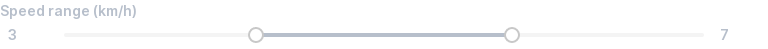# Range Slider¶

Use range sliders to allow picking a numeric range within a given range.

## Basic¶

Set `value=` to a `(start, end)` tuple to show a range slider.

The mode setting `mode='range'` is implied, and can be elided.

``````start, end = view(box('Speed range (km/h)', value=(3, 7)))
view(f'Your speed ranges between {start} and {end} km/h')
``````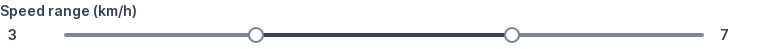## Set min value¶

Set `min=` to specify a minimum value.

``````start, end = view(box('Speed range (km/h)', value=(3, 7), min=3))
view(f'Your speed ranges between {start} and {end} km/h')
``````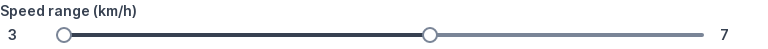## Set max value¶

Set `max=` to specify a maximum value.

``````start, end = view(box('Speed range (km/h)', value=(30, 70), max=100))
view(f'Your speed ranges between {start} and {end} km/h')
``````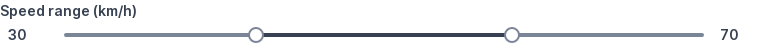## Set step¶

Set `step=` to specify how much to increment or decrement by.

The default step is `1`.

``````start, end = view(box('Speed range (km/h)', value=(2, 6), step=2))
view(f'Your speed ranges between {start} and {end} km/h')
``````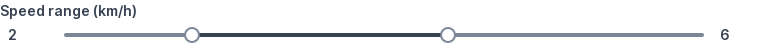## Set precision¶

Set `precision=` to specify how many decimal places the value should be rounded to.

The default is calculated based on the precision of step: - if step = 1, precision = 0 - if step = 0.42, precision = 2 - if step = 0.0042, precision = 4

``````start, end = view(box('Speed range (m/s)', value=(-0.4, 0.4), min=-2, max=2, step=0.2, precision=2))
view(f'Your speed ranges between {start} and {end} m/s')
``````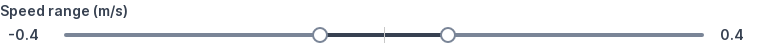## Combine min, max, step, precision¶

`min=`, `max=`, `step=` and `precision=` can be combined.

``````start, end = view(box('Speed range (km/h)', value=(30, 70), min=10, max=100, step=5))
view(f'Your speed ranges between {start} and {end} km/h')
``````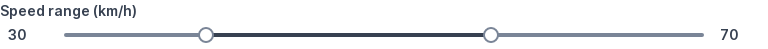## Set range¶

Set `range=` to a `(min, max)` tuple to restrict numeric inputs between two values.

This is a shorthand notation for setting `min=` and `max=` individually.

``````start, end = view(box('Speed range (km/h)', value=(30, 70), range=(10, 100)))
view(f'Your speed ranges between {start} and {end} km/h')
``````## Set range with step¶

Set `range=` to a `(min, max, step)` tuple to increment/decrement by steps other than `1`.

This is a shorthand notation for setting `min=`, `max=` and `step` individually.

``````start, end = view(box('Speed range (km/h)', value=(30, 70), range=(10, 100, 5)))
view(f'Your speed ranges between {start} and {end} km/h')
``````## Set range with precision¶

Set `range=` to a `(min, max, step)` tuple to increment/decrement by steps other than `1`. Setting `range=` to a `(min, max, step, precision)` tuple is shorthand for setting `min=`, `max=`, `step` and `precision` individually.

``````start, end = view(box('Speed range (m/s)', value=(-0.4, 0.4), range=(-2, 2, 0.2, 2)))
view(f'Your speed ranges between {start} and {end} m/s')
``````## Use zero-crossing range¶

Ranges can cross zero.

``````start, end = view(box('Speed range (m/s)', value=(-3, 3), range=(-5, 5)))
view(f'Your speed ranges between {start} and {end} m/s')
``````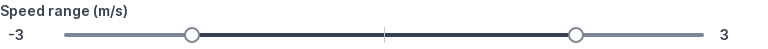## Set fractional steps¶

Steps can be fractional.

``````start, end = view(box('Speed range (m/s)', value=(-0.4, 0.4), range=(-2, 2, 0.2)))
view(f'Your speed ranges between {start} and {end} m/s')
``````## Handle changes immediately¶

Add `live` to `mode` to handle changes immediately.

``````start, end = 3, 7  # Starting values
while True:
start, end = view(
box('Speed range (km/h)', mode='live', value=(start, end)),
f'Your speed ranges between {start} and {end} km/h',
)
``````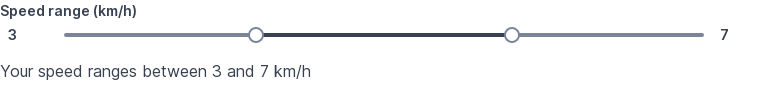## Disable¶

Set `disabled=True` to disable.

``````view(box('Speed range (km/h)', value=(3, 7), disabled=True))
``````